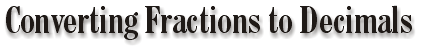Place Value

 Example: 432.567 The 2 is in the ones place. The 3 is in the tens place. The 4 is in the hundreds place. To the right of the decimal place… The 5 is in the tenths place. The 6 is in the hundredths place. The 7 is in the thousandths place.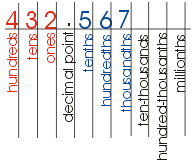The number is read as: "Four hundred thirty-two and five hundred sixty seven thousandths."

The Relationship Between Fractions and Decimals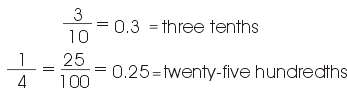The simplest of these conversions comes when the denominator is or can be very easily converted into a multiple of ten. This is the case for the previous two examples. In the next example, we cannot easily change the denominator, so we use the fraction bar as a division sign.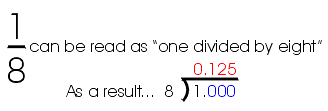In this division problem, note that the one is written as 1.000. In fact, we can add or "annex" as many zeros as we need to the right of the decimal point. This decimal that we found (.125) is called a terminating decimal because we can divide until we have a remainder of zero.

 In this division problem, note that we have annexed or added three zeros to help us divide. Each time we divide we get a remainder of one. The decimal that we found (.333…) is called a repeating decimal because no matter how many times we divide, our quotient will keep repeating.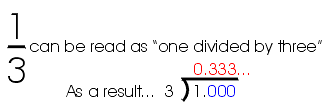Convert these fractions to decimals. Begin all decimals with a zero to the left. Ex. = 0.3 Round all repeating decimals to the nearest hundreth.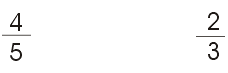ggggggggg                                     gggggggggggggggggggg

Decimals Main | Continue on to Comparing Fractions

 writer: Kevin Gallagher